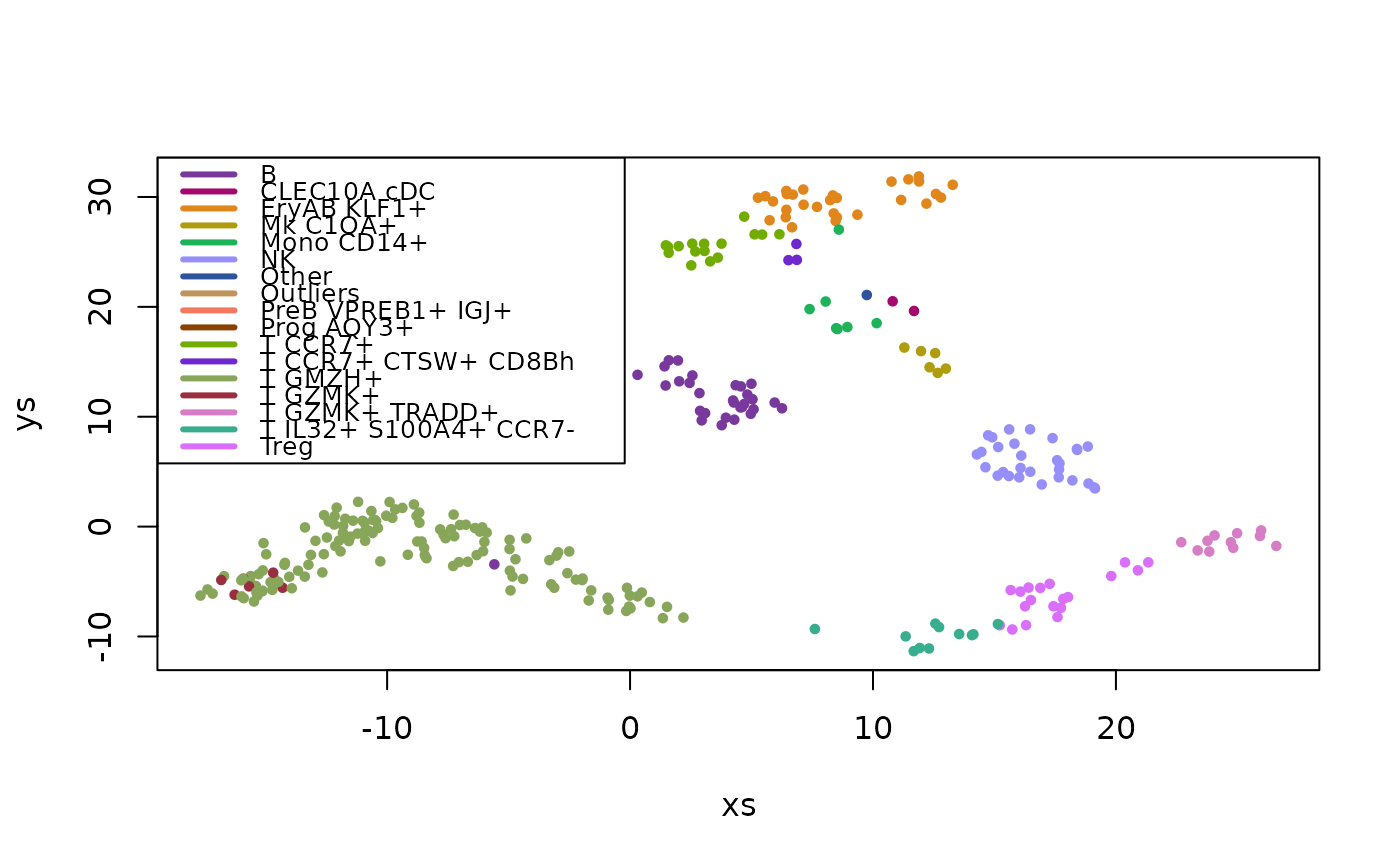This is a list with the following elements:

data(pbmc)

## Format

A list with the three elements described above.

## Details

umis - a matrix, containing ~1.5K metacells (rows), and for each one, the UMI count (# of detected RNA molecules) for each of ~600 different "feature" genes (columns).

types - a vector of cell type names assigned to each metacell using a supervised analysis pipeline.

umap - a matrix with 2 columns containing 2D UMAP x,y coordinates for each metacell.

## Examples

data(pbmc)
fractions <- pbmc$umis / rowSums(pbmc$umis)
log_fractions <- log2(fractions + 1e-5)
type_colors <- chameleon::data_colors(log_fractions, group=pbmc$types) plot(pbmc$umap, col=type_colors[pbmc\$types], pch=19, cex=0.6)
legend('topleft', legend=names(type_colors), col=type_colors, lty=1, lwd=3, cex=0.8)